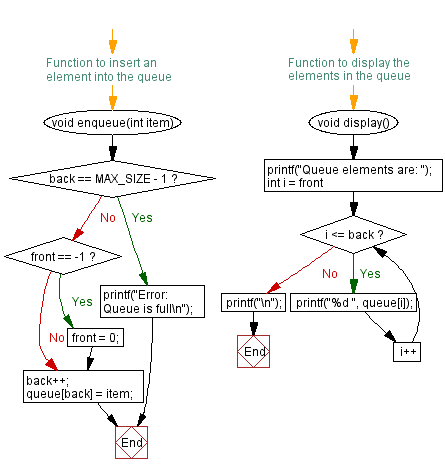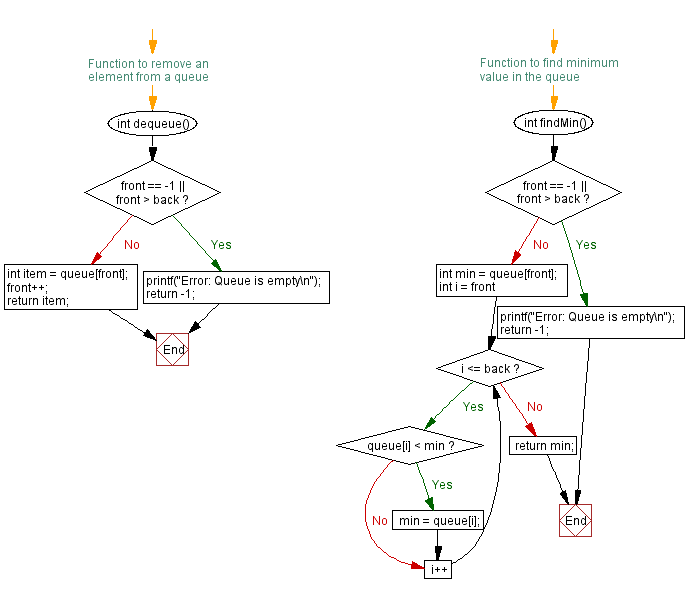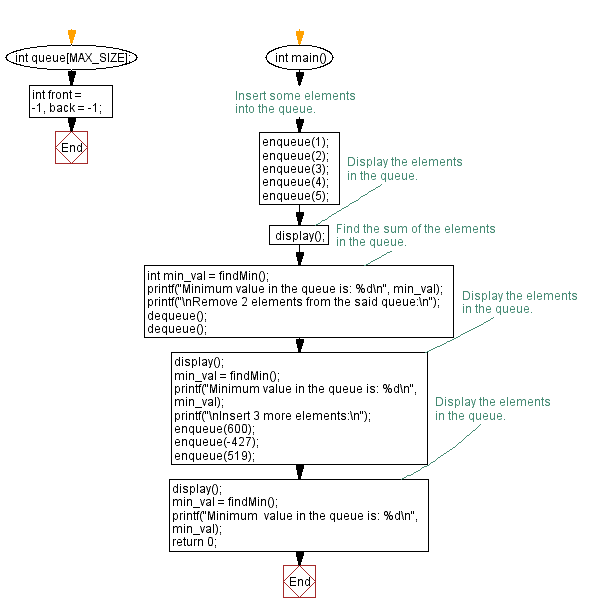﻿ C - Find the minimum element in a queue

# C Exercises: Find the minimum element in a queue

## C Queue: Exercise-10 with Solution

Write a C program to find the minimum element in a queue.

Sample Solution:

C Code:

``````#include <stdio.h>

#define MAX_SIZE 100

int queue[MAX_SIZE];
int front = -1, back = -1;

// Function to insert an element into the queue
void enqueue(int item) {
if (back == MAX_SIZE - 1) {
printf("Error: Queue is full\n");
return;
}
if (front == -1) {
front = 0;
}
back++;
queue[back] = item;
}

// Function to remove an element from a queue
int dequeue() {
if (front == -1 || front > back) {
printf("Error: Queue is empty\n");
return -1;
}
int item = queue[front];
front++;
return item;
}

// Function to find minimum value in the queue
int findMin() {
if (front == -1 || front > back) {
printf("Error: Queue is empty\n");
return -1;
}
int min = queue[front];
for (int i = front; i <= back; i++) {
if (queue[i] < min) {
min = queue[i];
}
}
return min;
}

// Function to display the elements in the queue
void display() {
printf("Queue elements are: ");
for (int i = front; i <= back; i++) {
printf("%d ", queue[i]);
}
printf("\n");
}

int main() {
// Insert some elements into the queue.
enqueue(1);
enqueue(2);
enqueue(3);
enqueue(4);
enqueue(5);
// Display the elements in the queue.
display();
// Find the sum of the elements in the queue.
int min_val = findMin();
printf("Minimum value in the queue is: %d\n", min_val);
printf("\nRemove 2 elements from the said queue:\n");
dequeue();
dequeue();
// Display the elements in the queue.
display();
min_val = findMin();
printf("Minimum value in the queue is: %d\n", min_val);
printf("\nInsert 3 more elements:\n");
enqueue(600);
enqueue(-427);
enqueue(519);
// Display the elements in the queue.
display();
min_val = findMin();
printf("Minimum  value in the queue is: %d\n", min_val);
return 0;;
}
```
```

Sample Output:

```Queue elements are: 1 2 3 4 5
Minimum value in the queue is: 1

Remove 2 elements from the said queue:
Queue elements are: 3 4 5
Minimum value in the queue is: 3

Insert 3 more elements:
Queue elements are: 3 4 5 600 -427 519
Minimum  value in the queue is: -427
```

Flowchart:C Programming Code Editor:

Have another way to solve this solution? Contribute your code (and comments) through Disqus.

What is the difficulty level of this exercise?

Test your Programming skills with w3resource's quiz.

﻿

## C Programming: Tips of the Day

C Programming - How do you pass a function as a parameter in C?

Declaration

A prototype for a function which takes a function parameter looks like the following:

```void func ( void (*f)(int) );
```

This states that the parameter f will be a pointer to a function which has a void return type and which takes a single int parameter. The following function (print) is an example of a function which could be passed to func as a parameter because it is the proper type:

```void print ( int x ) {
printf("%d\n", x);
}
```

Function Call

When calling a function with a function parameter, the value passed must be a pointer to a function. Use the function's name (without parentheses) for this:

```func(print);
```

would call func, passing the print function to it.

Function Body

As with any parameter, func can now use the parameter's name in the function body to access the value of the parameter. Let's say that func will apply the function it is passed to the numbers 0-4. Consider, first, what the loop would look like to call print directly:

```for ( int ctr = 0 ; ctr < 5 ; ctr++ ) {
print(ctr);
}
```

Since func's parameter declaration says that f is the name for a pointer to the desired function, we recall first that if f is a pointer then *f is the thing that f points to (i.e. the function print in this case). As a result, just replace every occurrence of print in the loop above with *f:

```void func ( void (*f)(int) ) {
for ( int ctr = 0 ; ctr < 5 ; ctr++ ) {
(*f)(ctr);
}
}
```

Ref : https://bit.ly/3skw9Um